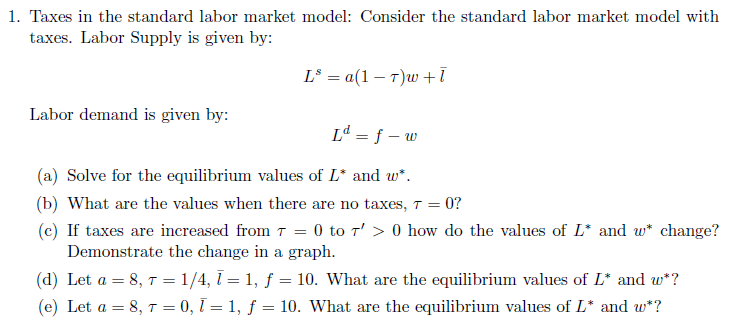### Standard Labor Market Model with TaxesExam Time –  I should make it clear that you’ll probably not get exactly (or all that close) to this problem. Thus focus on the steps you take toward a solution. Also, review Oct 18 lecture notes.Equilibrium labor supplied and demanded is the value ofsuch that.  What we do is set the labor demand curve equal to the labor supply curve, and solve for. You can then plug that into either equation, and it’ll provide the same value ofAnd this will also be equal toThis two messy equation, given values forwill be equal!If taxes, then, which implies thatStarting with wages:This makes theterm smaller, thus the denominator smaller, makingbigger.Therefore the wage workers insist upon and the wage employers must now pay is higher than without taxes. Note, though, that worker’s take-home wage is, after tax wages.For how Equilibrium Labor is affected by positive income tax – I’m going to use the equilibrium labor demand equation,since there is only oneterm appearing in it (while the labor supplied equationhas twoterms, which would make the discussion more complicated)This makes theterm smaller, making the denominator smaller in theterm.The smaller denominator makes theterm larger.With the negative sign in front of the biggerterm, this makessmaller.Graphically, you also want to show figure 7.4 on page 171.d.Wages:Labor Demand:Which should equal Labor Supplied:And we know that labor supplied equals labor demanded. So you should solve thethat is easiest mathematically. Or both if you really want that 100%e.Wages:Labor Demand:Which should equal Labor Supplied:Comments